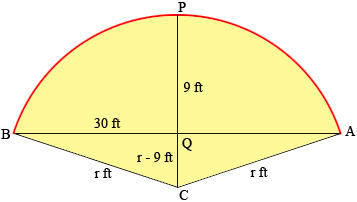SEARCH HOMEMath Central Quandaries & QueriesQuestion from Cory, a parent: A am wondering how to figure a falling arc. What I mean by this is: I have a chord length of 150'. From the left starting point, I know that 30' right of starting point is 9' in height. This would be the top of the arc. What is the arc in degrees and can anyone display an image to help me understand? Thank you in advance for your help.Hi Cory,

In my diagram (which is not to scale) the arc APB measures 150 feet and the radius of the circle is r feet.Triangle CQB is a right triangle and hence by Pythagoras theorem

302 + (r - 9)2 = r2

Solve for r.

The angle BCA subtends an arc of length 150 feet in a circle of radius r. What is the measure of the angle BCA?

I hope this helps,
PennyMath Central is supported by the University of Regina and The Pacific Institute for the Mathematical Sciences.